# Explore Fairness Metrics for Credit Scoring Model

This example shows how to calculate and display fairness metrics for two sensitive attributes. You can use these metrics to test data and the model for fairness and then determine the thresholds to apply for your situation. You can also use the metrics to understand the biases in your model, the levels of disparity between groups, and how to assess the fairness of the model.

### Fairness Metrics Calculations

Fairness metrics are a set of measures that enable you to detect the presence of bias in your data or model. Bias refers to the preference of one group over another group, implicitly or explicitly. When you detect bias in your data or model, you can decide to take action to mitigate the bias. Bias detection is a set of measures that enable you to see the presence of unfairness toward one group or another. Bias mitigation is a set of tools to reduce the amount of bias that occurs in the data or model for the current analysis.

A set of metrics exists for the data and a set of metrics also exists for the model. Group metrics measure information within the group, whereas bias metrics measure differences across groups. The example calculates two bias metrics (Statistical Parity Difference and Disparate Impact) and a group metric (group count) at the data level. In this example, you calculate four bias metrics and 11 group metrics at the model level.

Bias metrics:

• Statistical Parity Difference (SPD) measures the difference that the majority and protected classes receive a favorable outcome. This measure must be equal to 0 to be fair.

`$\mathrm{SPD}\text{\hspace{0.17em}}=\text{\hspace{0.17em}}\mathit{P}\left(\stackrel{ˆ}{\mathit{Y}}=1\text{\hspace{0.17em}}|\text{\hspace{0.17em}}\mathit{A}=\mathrm{minority}\right)-\mathit{P}\left(\stackrel{ˆ}{\mathit{Y}}=1\text{\hspace{0.17em}}|\text{\hspace{0.17em}}\mathit{A}=\mathrm{majority}\right),\mathrm{where}\text{\hspace{0.17em}}\stackrel{ˆ}{\mathit{Y}}\text{\hspace{0.17em}}\mathrm{are}\text{\hspace{0.17em}}\mathrm{the}\text{\hspace{0.17em}}\mathrm{model}\text{\hspace{0.17em}}\mathrm{predictions}\text{\hspace{0.17em}}\mathrm{and}\text{\hspace{0.17em}}\mathit{A}\text{\hspace{0.17em}}\mathrm{is}\text{\hspace{0.17em}}\mathrm{the}\text{\hspace{0.17em}}\mathrm{group}\text{\hspace{0.17em}}\mathrm{of}\text{\hspace{0.17em}}\mathrm{the}\text{\hspace{0.17em}}\mathrm{sensitive}\text{\hspace{0.17em}}\mathrm{attribute}.$`

• Disparate Impact (DI) compares the proportion of individuals that receive a favorable outcome for two groups, a majority group and a minority group. This measure must be equal to 1 to be fair.

`$\mathrm{DI}=\mathit{P}\left(\stackrel{ˆ}{\mathit{Y}}=1\text{\hspace{0.17em}}|\text{\hspace{0.17em}}\mathit{A}=\mathrm{minority}\right)\text{\hspace{0.17em}}/\text{\hspace{0.17em}}\mathit{P}\left(\stackrel{ˆ}{\mathit{Y}}=1\text{\hspace{0.17em}}|\text{\hspace{0.17em}}\mathit{A}=\mathrm{majority}\right),\mathrm{where}\text{\hspace{0.17em}}\stackrel{ˆ}{\mathit{Y}}\text{\hspace{0.17em}}\mathrm{are}\text{\hspace{0.17em}}\mathrm{the}\text{\hspace{0.17em}}\mathrm{model}\text{\hspace{0.17em}}\mathrm{predictions}\text{\hspace{0.17em}}\mathrm{and}\text{\hspace{0.17em}}\mathit{A}\text{\hspace{0.17em}}\mathrm{is}\text{\hspace{0.17em}}\mathrm{the}\text{\hspace{0.17em}}\mathrm{group}\text{\hspace{0.17em}}\mathrm{of}\text{\hspace{0.17em}}\mathrm{the}\text{\hspace{0.17em}}\mathrm{sensitive}\text{\hspace{0.17em}}\mathrm{attribute}.$`

• Equal Opportunity Difference (EOD) measures the deviation from the equality of opportunity, which means that the same proportion of each population receives the favorable outcome. This measure must be equal to 0 to be fair.

`$\mathrm{EOD}=\mathit{P}\left(\stackrel{ˆ}{\mathit{Y}}=1\text{\hspace{0.17em}}|\text{\hspace{0.17em}}\mathit{A}=\text{\hspace{0.17em}}\mathrm{minority},\mathit{Y}=1\right)-\mathit{P}\left(\stackrel{ˆ}{\mathit{Y}}=1\text{\hspace{0.17em}}|\text{\hspace{0.17em}}\mathit{A}=\text{\hspace{0.17em}}\mathrm{majority},\mathit{Y}=1\right),\mathrm{where}\text{\hspace{0.17em}}\stackrel{ˆ}{\mathit{Y}}\text{\hspace{0.17em}}\mathrm{are}\text{\hspace{0.17em}}\mathrm{the}\text{\hspace{0.17em}}\mathrm{model}\text{\hspace{0.17em}}\mathrm{predictions},\text{\hspace{0.17em}}\mathit{A}\text{\hspace{0.17em}}\mathrm{is}\text{\hspace{0.17em}}\mathrm{the}\text{\hspace{0.17em}}\mathrm{group}\text{\hspace{0.17em}}\mathrm{of}\text{\hspace{0.17em}}\mathrm{the}\text{\hspace{0.17em}}\mathrm{sensitive}\text{\hspace{0.17em}}\mathrm{attribute},\text{\hspace{0.17em}}\mathrm{and}\text{\hspace{0.17em}}\mathit{Y}\text{\hspace{0.17em}}\mathrm{are}\text{\hspace{0.17em}}\mathrm{the}\text{\hspace{0.17em}}\mathrm{true}\text{\hspace{0.17em}}\mathrm{labels}.$`

• Average Absolute Odds Difference (AAOD) measures bias by using the false positive rate and true positive rate. This measure must be equal to 0 to be fair.

`$\begin{array}{l}\mathrm{AAOD}=\frac{1}{2}\left[|\mathit{FP}{\mathit{R}}_{\mathit{A}=\mathit{minority}}-\mathit{FP}{\mathit{R}}_{\mathit{A}=\mathit{majority}}|+|\mathit{TP}{\mathit{R}}_{\mathit{A}=\mathit{minority}}-\mathit{TP}{\mathit{R}}_{\mathit{A}=\mathit{majority}}|\right],\\ \mathrm{where}\text{\hspace{0.17em}}\mathit{A}\text{\hspace{0.17em}}\mathrm{is}\text{\hspace{0.17em}}\mathrm{the}\text{\hspace{0.17em}}\mathrm{group}\text{\hspace{0.17em}}\mathrm{of}\text{\hspace{0.17em}}\mathrm{the}\text{\hspace{0.17em}}\mathrm{sensitive}\text{\hspace{0.17em}}\mathrm{attribute}.\end{array}$`

Group metrics:

• Group Count is the number of individuals in the group.

• True Positive Rate (TPR) is the sensitivity.

`$\begin{array}{l}\mathrm{TPR}=\text{\hspace{0.17em}}\frac{\mathrm{TP}}{\left(\mathrm{TP}+\mathrm{FN}\right)},\\ \mathrm{where}\text{\hspace{0.17em}}\mathrm{TP}\text{\hspace{0.17em}}\mathrm{is}\text{\hspace{0.17em}}\mathrm{true}\text{\hspace{0.17em}}\mathrm{positive}\text{\hspace{0.17em}}\mathrm{and}\text{\hspace{0.17em}}\mathrm{FN}\text{\hspace{0.17em}}\mathrm{is}\text{\hspace{0.17em}}\mathrm{false}\text{\hspace{0.17em}}\mathrm{negative}.\end{array}$`

• True Negative Rate (TNR) is the specificity or selectivity.

`$\begin{array}{l}\mathrm{TNR}=\text{\hspace{0.17em}}\frac{\mathrm{TN}}{\left(\mathrm{TN}+\mathrm{FP}\right)},\\ \mathrm{where}\text{\hspace{0.17em}}\mathrm{TN}\text{\hspace{0.17em}}\mathrm{is}\text{\hspace{0.17em}}\mathrm{true}\text{\hspace{0.17em}}\mathrm{negative}\text{\hspace{0.17em}}\mathrm{and}\text{\hspace{0.17em}}\mathrm{FP}\text{\hspace{0.17em}}\mathrm{is}\text{\hspace{0.17em}}\mathrm{false}\text{\hspace{0.17em}}\mathrm{positive}.\end{array}$`

• False Positive Rate (FPR) is the Type-I error.

`$\mathrm{FPR}=\text{\hspace{0.17em}}\frac{\mathrm{FP}}{\left(\mathrm{FP}+\mathrm{TN}\right)}$`

• False Negative Rate (FNR) is the Type-II error.

`$\mathrm{FNR}=\text{\hspace{0.17em}}\frac{\mathrm{FN}}{\left(\mathrm{FN}+\mathrm{TP}\right)}$`

• False Discovery Rate (FDR) is the ratio of the number of false positive results to the number of total positive test results.

`$\mathrm{FDR}=\text{\hspace{0.17em}}\frac{\mathrm{FP}}{\left(\mathrm{FP}+\mathrm{TP}\right)}$`

• False Omission Rate (FOR) is the ratio of the number of individuals with a negative predicted value for which the true label is positive.

`$\mathrm{FOR}=\text{\hspace{0.17em}}\frac{\mathrm{FN}}{\left(\mathrm{FN}+\mathrm{TN}\right)}$`

• Positive Predictive Value (PPV) is the ratio of the number of true positives to the number of true positives and false positives.

`$\mathrm{PPV}=\text{\hspace{0.17em}}\frac{\mathrm{TP}}{\left(\mathrm{TP}+\mathrm{FP}\right)}$`

• Negative Predictive Value (NPV) is the ratio of the number of true negatives to the number of true positives and false positives.

`$\mathrm{NPV}=\text{\hspace{0.17em}}\frac{\mathrm{TN}}{\left(\mathrm{TN}+\mathrm{FN}\right)}$`

• Acceptance Rate (AR) is the ratio of the number of false and true positives to the total observations.

`$\mathrm{AR}=\text{\hspace{0.17em}}\frac{\left(\mathrm{FP}+\mathrm{TP}\right)}{\left(\mathrm{TN}+\mathrm{TP}+\mathrm{FN}+\mathrm{FP}\right)}$`

• Accuracy (ACC) is the ratio of the number of true negatives and true positives to the total observations.

`$\mathrm{ACC}=\text{\hspace{0.17em}}\frac{\left(\mathrm{TN}+\mathrm{TP}\right)}{\left(\mathrm{TN}+\mathrm{TP}+\mathrm{FN}+\mathrm{FP}\right)}$`

The example focuses on bias detection in credit card data and explores bias metrics and group metrics based on the sensitive attributes of customer age and residential status. The data contains the residential status as a categorical variable and the customer age as a numeric variable. To create predictions and analyze the data for fairness, you group the customer age variable into bins.

### Visualize Sensitive Attributes in Credit Card Data

Load the credit card data set. Group the customer age into bins. Use the `discretize` function for a numeric variable to create groups that identify age groups of interest for comparison on fairness. Retrieve the counts for both sensitive attributes of customer age and residential status.

```load CreditCardData.mat AgeGroup = discretize(data.CustAge,[min(data.CustAge) 30 45 60 max(data.CustAge)], ... 'categorical',{'Age <= 30','30 < Age <= 45','45 < Age <= 60','Age > 60'}); data = addvars(data,AgeGroup,'After','CustAge'); gs_data_ResStatus = groupsummary(data,{'ResStatus','status'}); gs_data_AgeGroup = groupsummary(data,{'AgeGroup','status'}); ```

Plot the count of customers who have defaulted on their credit card payments and who have not defaulted by age.

```Attribute =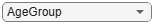"AgeGroup"; figure bar(unique(data.(Attribute)), ... [eval("gs_data_"+Attribute+".GroupCount(1:2:end)"), ... eval("gs_data_"+Attribute+".GroupCount(2:2:end)")]'); title(Attribute +" True Counts"); ylabel('Counts') legend({'Nondefaults','Defaults'})```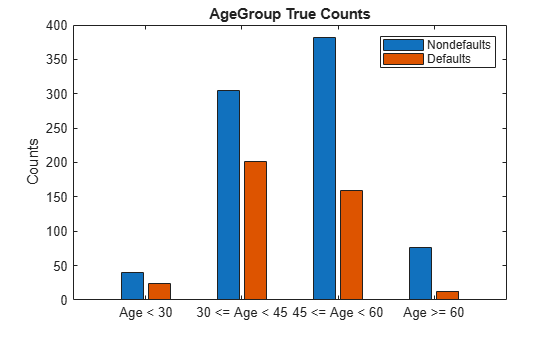### Calculate Fairness Metrics for Data

Calculate fairness metrics for the residential status data. The `fairnessMetrics` function returns the result as two tables, one for bias metrics and the other for group metrics. Bias metrics take into account two classes (the majority and minority) at a time, while group metrics are within the individual group. In the data set, if we use residential status as the sensitive attribute, then the `Home Owner` group is the majority class because this class contains the largest number of individuals. Based on the SPD and DI metrics, the data set does not show a significant presence of bias for residential status.

```[dataBiasMetrics_ResStatus,dataGroupMetrics_ResStatus] = fairnessMetrics(data.ResStatus, ... data.status)```
```dataBiasMetrics_ResStatus=3×3 table Group StatisticalParityDifference DisparateImpact ____________ ___________________________ _______________ "Home Owner" 0 1 "Tenant" 0.025752 1.0789 "Other" -0.038525 0.88203 ```
```dataGroupMetrics_ResStatus=3×2 table Group GroupCount ____________ __________ "Home Owner" 542 "Tenant" 474 "Other" 184 ```

Calculate fairness metrics for the customer age data. In the data set, the age group between 45 and 60 is the majority class because this class contains the largest number of individuals. Compared to the residential status, based on the SPD and DI metrics, the age group that is greater than 60 shows a slightly larger presence of bias.

```[dataBiasMetrics_AgeGroup,dataGroupMetrics_AgeGroup] = fairnessMetrics(data.AgeGroup, ... data.status)```
```dataBiasMetrics_AgeGroup=4×3 table Group StatisticalParityDifference DisparateImpact ________________ ___________________________ _______________ "Age <= 30" 0.0811 1.2759 "30 < Age <= 45" 0.10333 1.3516 "45 < Age <= 60" 0 1 "Age > 60" -0.14783 0.497 ```
```dataGroupMetrics_AgeGroup=4×2 table Group GroupCount ________________ __________ "Age <= 30" 64 "30 < Age <= 45" 506 "45 < Age <= 60" 541 "Age > 60" 89 ```

### Create Credit Scorecard Model and Generate Predictions

Create a credit scorecard model using the `creditscorecard` function. Perform automatic binning of the predictors using the `autobinning` function. Fit a logistic regression model to the Weight of Evidence data using the `fitmodel` function. Store the predictor names and corresponding coefficients in the credit scorecard model.

```PredictorVars = setdiff(data.Properties.VariableNames, ... {'AgeGroup','CustID','status'}); sc = creditscorecard(data,'IDVar','CustID', ... 'PredictorVars',PredictorVars); sc = autobinning(sc); sc = fitmodel(sc);```
```1. Adding CustIncome, Deviance = 1490.8527, Chi2Stat = 32.588614, PValue = 1.1387992e-08 2. Adding TmWBank, Deviance = 1467.1415, Chi2Stat = 23.711203, PValue = 1.1192909e-06 3. Adding AMBalance, Deviance = 1455.5715, Chi2Stat = 11.569967, PValue = 0.00067025601 4. Adding EmpStatus, Deviance = 1447.3451, Chi2Stat = 8.2264038, PValue = 0.0041285257 5. Adding CustAge, Deviance = 1441.994, Chi2Stat = 5.3511754, PValue = 0.020708306 6. Adding ResStatus, Deviance = 1437.8756, Chi2Stat = 4.118404, PValue = 0.042419078 7. Adding OtherCC, Deviance = 1433.707, Chi2Stat = 4.1686018, PValue = 0.041179769 Generalized linear regression model: status ~ [Linear formula with 8 terms in 7 predictors] Distribution = Binomial Estimated Coefficients: Estimate SE tStat pValue ________ ________ ______ __________ (Intercept) 0.70239 0.064001 10.975 5.0538e-28 CustAge 0.60833 0.24932 2.44 0.014687 ResStatus 1.377 0.65272 2.1097 0.034888 EmpStatus 0.88565 0.293 3.0227 0.0025055 CustIncome 0.70164 0.21844 3.2121 0.0013179 TmWBank 1.1074 0.23271 4.7589 1.9464e-06 OtherCC 1.0883 0.52912 2.0569 0.039696 AMBalance 1.045 0.32214 3.2439 0.0011792 1200 observations, 1192 error degrees of freedom Dispersion: 1 Chi^2-statistic vs. constant model: 89.7, p-value = 1.4e-16 ```

Display unscaled points for predictors retained in the model using the `displaypoints` function.

`pointsinfo = displaypoints(sc)`
```pointsinfo=37×3 table Predictors Bin Points ______________ ________________ _________ {'CustAge' } {'[-Inf,33)' } -0.15894 {'CustAge' } {'[33,37)' } -0.14036 {'CustAge' } {'[37,40)' } -0.060323 {'CustAge' } {'[40,46)' } 0.046408 {'CustAge' } {'[46,48)' } 0.21445 {'CustAge' } {'[48,58)' } 0.23039 {'CustAge' } {'[58,Inf]' } 0.479 {'CustAge' } {'<missing>' } NaN {'ResStatus' } {'Tenant' } -0.031252 {'ResStatus' } {'Home Owner' } 0.12696 {'ResStatus' } {'Other' } 0.37641 {'ResStatus' } {'<missing>' } NaN {'EmpStatus' } {'Unknown' } -0.076317 {'EmpStatus' } {'Employed' } 0.31449 {'EmpStatus' } {'<missing>' } NaN {'CustIncome'} {'[-Inf,29000)'} -0.45716 ⋮ ```

For details about creating a more in depth credit scoring model, see the Binning Explorer Case Study Example.

Calculate the probability of default for the credit scorecard model using the `probdefault` function. Define the threshold for the probability of default as 0.35. Create an array of predictions where each value is greater than the threshold.

```pd = probdefault(sc); threshold = 0.35; predictions = double(pd>threshold);```

Add the resulting predictions to the `data` output table. To calculate bias metrics, you can set aside a set of validation data. Retrieve the counts for the residential status and customer age predictions. Plot the customer age predictions.

```data = addvars(data,predictions,'After','status'); gs_predictions_ResStatus = groupsummary(data,{'ResStatus','predictions'}, ... 'IncludeEmptyGroups',true); gs_predictions_AgeGroup = groupsummary(data,{'AgeGroup','predictions'}, ... 'IncludeEmptyGroups',true); Attribute ="AgeGroup"; figure bar(unique(data.(Attribute)), ... [eval("gs_predictions_"+Attribute+".GroupCount(1:2:end)"), ... eval("gs_predictions_"+Attribute+".GroupCount(2:2:end)")]'); title(Attribute +" Prediction Counts"); ylabel('Counts') legend({'Nondefaults','Defaults'})```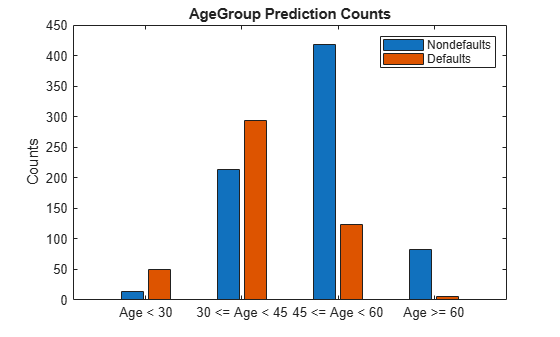### Calculate and Visualize Fairness Metrics for Credit Scorecard Model

Calculate model bias and group metrics for residential status. For the DI model metric, the commonly used range to assess fairness is between 0.8 and 1.2 . A value of less than 0.8 indicates the presence of bias. However, a value greater than 1.2 indicates that something is incorrect and additional investigation might be required. The model bias metrics in this example show a greater effect on fairness than the data bias metrics. After the model has been fitted, the negative SPD and EOD values mean that the `Other` group shows a slight presence of bias. In the group metrics, the FPR group metric of 39.7% is higher for tenants than home owners, which means that tenants are more likely to be falsely labeled as defaults. The FDR, FOR, PPV, and NPV group metrics show a very minimal presence of bias.

```[biasMetrics_ResStatus,groupMetrics_ResStatus] = fairnessMetrics(data.ResStatus, ... data.status,predictions)```
```biasMetrics_ResStatus=3×5 table Group StatisticalParityDifference DisparateImpact EqualOpportunityDifference AverageAbsoluteOddsDifference ____________ ___________________________ _______________ __________________________ _____________________________ "Home Owner" 0 1 0 0 "Tenant" 0.10173 1.2743 0.1136 0.1007 "Other" -0.11541 0.68878 -0.082081 0.10042 ```
```groupMetrics_ResStatus=3×16 table Group GroupCount TP TN FP FN TPR TNR FPR FNR FDR FOR PPV NPV AcceptanceRate Accuracy ____________ __________ ___ ___ ___ __ _______ _______ _______ _______ _______ _______ _______ _______ ______________ ________ "Home Owner" 542 88 252 113 89 0.49718 0.69041 0.30959 0.50282 0.56219 0.261 0.43781 0.739 0.37085 0.62731 "Tenant" 474 102 185 122 65 0.61078 0.60261 0.39739 0.38922 0.54464 0.26 0.45536 0.74 0.47257 0.60549 "Other" 184 22 106 25 31 0.41509 0.80916 0.19084 0.58491 0.53191 0.22628 0.46809 0.77372 0.25543 0.69565 ```

Calculate model bias and group metrics for customer age. For model metrics SPD, DI, EOD, and AAOD, the 30 and under group has the greatest variance from the majority class and might require further investigation. Further, the age group over 60 shows the presence of bias based on the negative SPD and EOD values and the very low DI value. Also, based on the DI metrics, additional model bias mitigation might be required.

In the group metrics, the FPR group metric of 80% is much higher for the 30 and under group than the majority class, which means that those individuals whose age is 30 and under are more likely to be falsely labeled as defaults. The FDR group metric of 83.3% is much higher for the over 60 group than the majority class, which means that 83.3% of individuals whose age is over 60 and identified as defaults by the model are false positives. The `Accuracy` metric shows the highest accuracy for the over 60 group at 80.9%.

```[biasMetrics_AgeGroup,groupMetrics_AgeGroup] = fairnessMetrics(data.AgeGroup, ... data.status,predictions)```
```biasMetrics_AgeGroup=4×5 table Group StatisticalParityDifference DisparateImpact EqualOpportunityDifference AverageAbsoluteOddsDifference ________________ ___________________________ _______________ __________________________ _____________________________ "Age <= 30" 0.55389 3.4362 0.41038 0.51487 "30 < Age <= 45" 0.35169 2.5469 0.35192 0.3381 "45 < Age <= 60" 0 1 0 0 "Age > 60" -0.15994 0.29652 -0.2627 0.18877 ```
```groupMetrics_AgeGroup=4×16 table Group GroupCount TP TN FP FN TPR TNR FPR FNR FDR FOR PPV NPV AcceptanceRate Accuracy ________________ __________ ___ ___ ___ ___ ________ _______ ________ _______ _______ _______ _______ _______ ______________ ________ "Age <= 30" 64 18 8 32 6 0.75 0.2 0.8 0.25 0.64 0.42857 0.36 0.57143 0.78125 0.40625 "30 < Age <= 45" 506 139 151 154 62 0.69154 0.49508 0.50492 0.30846 0.5256 0.29108 0.4744 0.70892 0.57905 0.57312 "45 < Age <= 60" 541 54 313 69 105 0.33962 0.81937 0.18063 0.66038 0.56098 0.2512 0.43902 0.7488 0.22736 0.67837 "Age > 60" 89 1 71 5 12 0.076923 0.93421 0.065789 0.92308 0.83333 0.14458 0.16667 0.85542 0.067416 0.80899 ```

Plot these bias metrics for the two sensitive attributes using the plotMetrics function: SPD, DI, EOD, and AAOD.

`plotMetrics(biasMetrics_ResStatus,biasMetrics_AgeGroup);`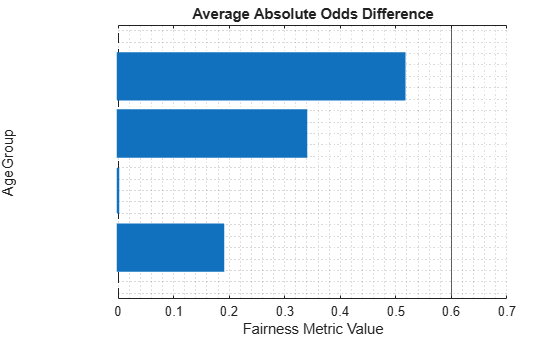Choose the bias metric and plot it for each sensitive attribute. This code selects AAOD by default. The resulting plots show the metric values for residential status and customer age.

```BiasMetric =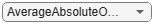"AverageAbsoluteOddsDifference"; plotMetrics(biasMetrics_ResStatus(:,["Group",BiasMetric]), ... biasMetrics_AgeGroup(:,["Group",BiasMetric]))```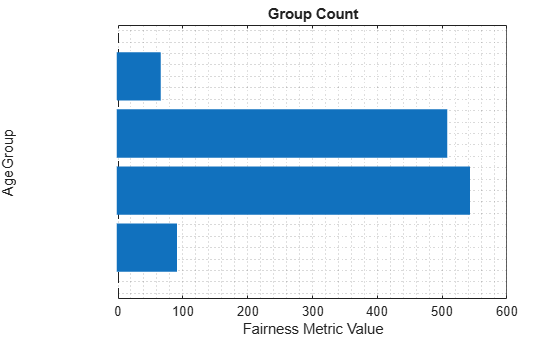For the same sensitive attributes, choose the group metric and plot it for each sensitive attribute. This code selects the group count by default. The resulting plots show the metric values for residential status and customer age.

```GroupMetric ="GroupCount"; plotMetrics(groupMetrics_ResStatus(:,["Group",GroupMetric]), ... groupMetrics_AgeGroup(:,["Group",GroupMetric]))```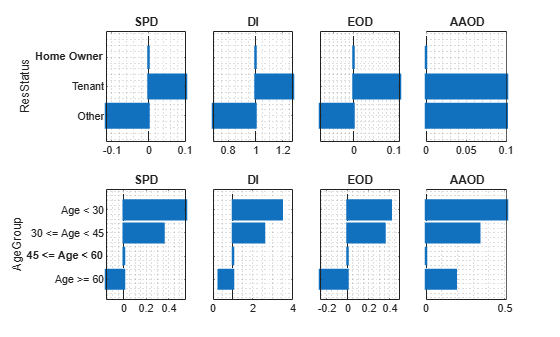Bias preserving metrics seek to keep the historic performance in the outputs of a target model with equivalent error rates for each group as shown in the training data. These metrics do not alter the status quo that exists in society. A fairness metric is classified as bias preserving when a perfect classifier exactly satisfies the metric. In contrast, bias transforming metrics require the explicit decision regarding which biases the system should exhibit. These metrics do not accept the status quo and acknowledge that protected groups start from different points that are not equal. The main difference between these two types of metrics is that most bias transforming metrics are satisfied by matching decision rates between groups, whereas bias preserving metrics require matching error rates instead. To assess the fairness of a decision-making system, use both bias preserving and transforming metrics to create the broadest possible view of the bias in the system.

Evaluating whether a metric is bias preserving is straightforward with a perfect classifier. In the absence of a perfect classifier, you can substitute the predictions with the classifier response and observe if the formula is trivially true. EOD and AAOD are bias preserving metrics because they have no variance; however, SPD and DI are bias transforming metrics as they show a variance from the majority classes.

`biasMetrics_ResStatus1 = fairnessMetrics(data.ResStatus,data.status,data.status)`
```biasMetrics_ResStatus1=3×5 table Group StatisticalParityDifference DisparateImpact EqualOpportunityDifference AverageAbsoluteOddsDifference ____________ ___________________________ _______________ __________________________ _____________________________ "Home Owner" 0 1 0 0 "Tenant" 0.025752 1.0789 0 0 "Other" -0.038525 0.88203 0 0 ```
`biasMetrics_AgeGroup1 = fairnessMetrics(data.AgeGroup,data.status,data.status)`
```biasMetrics_AgeGroup1=4×5 table Group StatisticalParityDifference DisparateImpact EqualOpportunityDifference AverageAbsoluteOddsDifference ________________ ___________________________ _______________ __________________________ _____________________________ "Age <= 30" 0.0811 1.2759 0 0 "30 < Age <= 45" 0.10333 1.3516 0 0 "45 < Age <= 60" 0 1 0 0 "Age > 60" -0.14783 0.497 0 0 ```

### References

1. Schmidt, Nicolas, Sue Shay, Steve Dickerson, Patrick Haggerty, Arjun R. Kannan, Kostas Kotsiopoulos, Raghu Kulkarni, Alexey Miroshnikov, Kate Prochaska, Melanie Wiwczaroski, Benjamin Cox, Patrick Hall, and Josephine Wang. Machine Learning: Considerations for Fairly and Transparently Expanding Access to Credit. Mountain View, CA: H2O.ai, Inc., July 2020.

2. Mehrabi, Ninareh, et al. “A Survey on Bias and Fairness in Machine Learning.” ArXiv:1908.09635 [Cs], Sept. 2019. arXiv.org, https://arxiv.org/abs/1908.09635.

3. Saleiro, Pedro, et al. “Aequitas: A Bias and Fairness Audit Toolkit.” ArXiv:1811.05577 [Cs], Apr. 2019. arXiv.org, https://arxiv.org/abs/1811.05577.

4. Wachter, Sandra, et al. Bias Preservation in Machine Learning: The Legality of Fairness Metrics Under EU Non-Discrimination Law. SSRN Scholarly Paper, ID 3792772, Social Science Research Network, 15 Jan. 2021. papers.ssrn.com, https://papers.ssrn.com/abstract=3792772.

### Local Helper Function

This code creates the `fairnessMetrics` and `plotMetrics` functions.

```function [biasMetrics,groupMetrics] = fairnessMetrics(Groups, ... Actual,varargin) % fairnessMetrics uses a sensitive attribute, true labels, and predictions % to calculate bias metrics and group metrics for the data and model. if isempty(varargin) % Calculate data metrics. [data_gs,cats,counts] = groupsummary(Actual,Groups,'mean'); [~,maxInd] = max(counts); numGroups = numel(cats); groupMetrics = array2table(zeros(numGroups,1)); groupMetrics.Properties.VariableNames = {'GroupCount'}; biasMetrics = array2table(zeros(numGroups,2)); % fairnessMetrics calculates only the Statistical Parity Difference and % Disparate Impact bias metrics. biasMetrics.Properties.VariableNames = {'StatisticalParityDifference', ... 'DisparateImpact'}; Group = string(cats); groupMetrics = addvars(groupMetrics,Group,'Before','GroupCount'); biasMetrics = addvars(biasMetrics,Group,'Before', ... 'StatisticalParityDifference'); biasMetrics.StatisticalParityDifference = data_gs - data_gs(maxInd); biasMetrics.DisparateImpact = data_gs ./ data_gs(maxInd); else % Calculate model metrics with predictions. Predicted = varargin{1}; [predicted_gs,cats,counts] = groupsummary(Predicted,Groups,'mean'); [~,maxInd] = max(counts); MajorityClass = string(cats(maxInd)); numGroups = numel(cats); % Set up output for group metrics. groupMetrics = array2table(zeros(numGroups,15)); groupMetrics.Properties.VariableNames = {'GroupCount','TP','TN','FP', ... 'FN','TPR','TNR','FPR','FNR','FDR','FOR','PPV','NPV', ... 'AcceptanceRate','Accuracy'}; % Set up output for bias metrics. biasMetrics = array2table(zeros(numGroups, 4)); biasMetrics.Properties.VariableNames = {'StatisticalParityDifference', ... 'DisparateImpact','EqualOpportunityDifference', ... 'AverageAbsoluteOddsDifference'}; Group = string(cats); groupMetrics = addvars(groupMetrics,Group,'Before','GroupCount'); biasMetrics = addvars(biasMetrics,Group,'Before', ... 'StatisticalParityDifference'); for i = 1:numGroups c_mat = confusionmat(Actual(Groups==cats(i)),Predicted(Groups==cats(i))); % True Positive TP = c_mat(2,2); % True Negative TN = c_mat(1,1); % False Positive FP = c_mat(1,2); % False Negative FN = c_mat(2,1); % Set the values for group metrics. groupMetrics{i,'TP'} = TP; groupMetrics{i,'FP'} = FP; groupMetrics{i,'TN'} = TN; groupMetrics{i,'FN'} = FN; groupMetrics{i,'TPR'} = TP / (TP + FN); % True Positive Rate, Sensitivity (1-TypeII) groupMetrics{i,'TNR'} = TN / (TN + FP); % True Negative Rate, Specificity or Selectivity (1-TypeI) groupMetrics{i,'FPR'} = FP / (FP + TN); % False Positive Rate, Type-I error groupMetrics{i,'FNR'} = FN / (FN + TP); % False Negative Rate, Type-II error groupMetrics{i,'FDR'} = FP / (FP + TP); % False Discovery Rate groupMetrics{i,'FOR'} = FN / (FN + TN); % False Ommission Rate groupMetrics{i,'PPV'} = TP / (TP + FP); % Positive Predictive Value (1-FDR) groupMetrics{i,'NPV'} = TN / (TN + FN); % Negative Predictive Value (1-FOR) % Calculate Acceptance Rate and Accuracy. groupMetrics{i,'AcceptanceRate'} = (FP + TP) / (TN + TP + FN + FP); groupMetrics{i,'Accuracy'} = (TN + TP) / (TN + TP + FN + FP); end for i = 1:numGroups % Equal Opportunity Difference biasMetrics{i,'EqualOpportunityDifference'} = groupMetrics{i,'TPR'} - ... groupMetrics{groupMetrics.Group==MajorityClass,'TPR'}; % Average Absolute Odds Difference biasMetrics{i,'AverageAbsoluteOddsDifference'} = 0.5*(abs(groupMetrics{i,'FPR'} - ... groupMetrics{groupMetrics.Group==MajorityClass,'FPR'}) + ... abs(groupMetrics{i,'TPR'} - groupMetrics{groupMetrics.Group==MajorityClass,'TPR'})); end biasMetrics.StatisticalParityDifference = predicted_gs - predicted_gs(maxInd); % Statistical Parity Difference biasMetrics.DisparateImpact = predicted_gs ./ predicted_gs(maxInd); % Disparate Impact end groupMetrics.GroupCount = counts; end function plotMetrics(ResStatusMetrics,AgeGroupMetrics) % plotMetrics creates plots that show the specified metrics in % a series of subplots. if width(ResStatusMetrics)==5 % Create subplot with all metrics. tiledlayout(2,4); % Residential status subplots nexttile barh(ResStatusMetrics{:,2}); yticklabels(ResStatusMetrics.Group) ax = gca; ax.YDir = 'reverse'; ylabel('ResStatus'); title('SPD'); % Statistical Parity Difference grid on nexttile barh(ResStatusMetrics{:,3}); ax = gca; ax.YDir = 'reverse'; ax.YTickLabel = ''; title('DI'); % Discrete Impact grid on nexttile barh(ResStatusMetrics{:,4}); ax = gca; ax.YDir = 'reverse'; ax.YTickLabel = ''; title('EOD'); % Equal Opportunity Difference grid on nexttile barh(ResStatusMetrics{:,5}); ax = gca; ax.YDir = 'reverse'; ax.YTickLabel = ''; title('AAOD'); % Average Absolute Odds Difference grid on nexttile barh(AgeGroupMetrics{:,2}); % Customer age subplots yticklabels(AgeGroupMetrics.Group) ax = gca; ax.YDir = 'reverse'; ylabel('AgeGroup'); title('SPD'); grid on nexttile barh(AgeGroupMetrics{:,3}); ax = gca; ax.YDir = 'reverse'; ax.YTickLabel = ''; title('DI'); grid on nexttile barh(AgeGroupMetrics{:,4}); ax = gca; ax.YDir = 'reverse'; ax.YTickLabel = ''; title('EOD'); grid on nexttile barh(AgeGroupMetrics{:,5}); ax = gca; ax.YDir = 'reverse'; ax.YTickLabel = ''; title('AAOD'); grid on else % Create subplot with just one metric for each sensitive attribute. tiledlayout(2,1) nexttile barh(ResStatusMetrics{:,2}); yticklabels(ResStatusMetrics.Group) % Residential status group metric ax(1) = gca; ax(1).YDir = 'reverse'; ylabel('ResStatus'); title(ResStatusMetrics.Properties.VariableNames{2}); grid on nexttile barh(AgeGroupMetrics{:,2}); yticklabels(AgeGroupMetrics.Group) % Customer age group metric ax(2) = gca; ax(2).YDir = 'reverse'; ylabel('AgeGroup'); title(AgeGroupMetrics.Properties.VariableNames{2}); grid on linkaxes(ax,'x') % Link x-axes for both subplots end end```